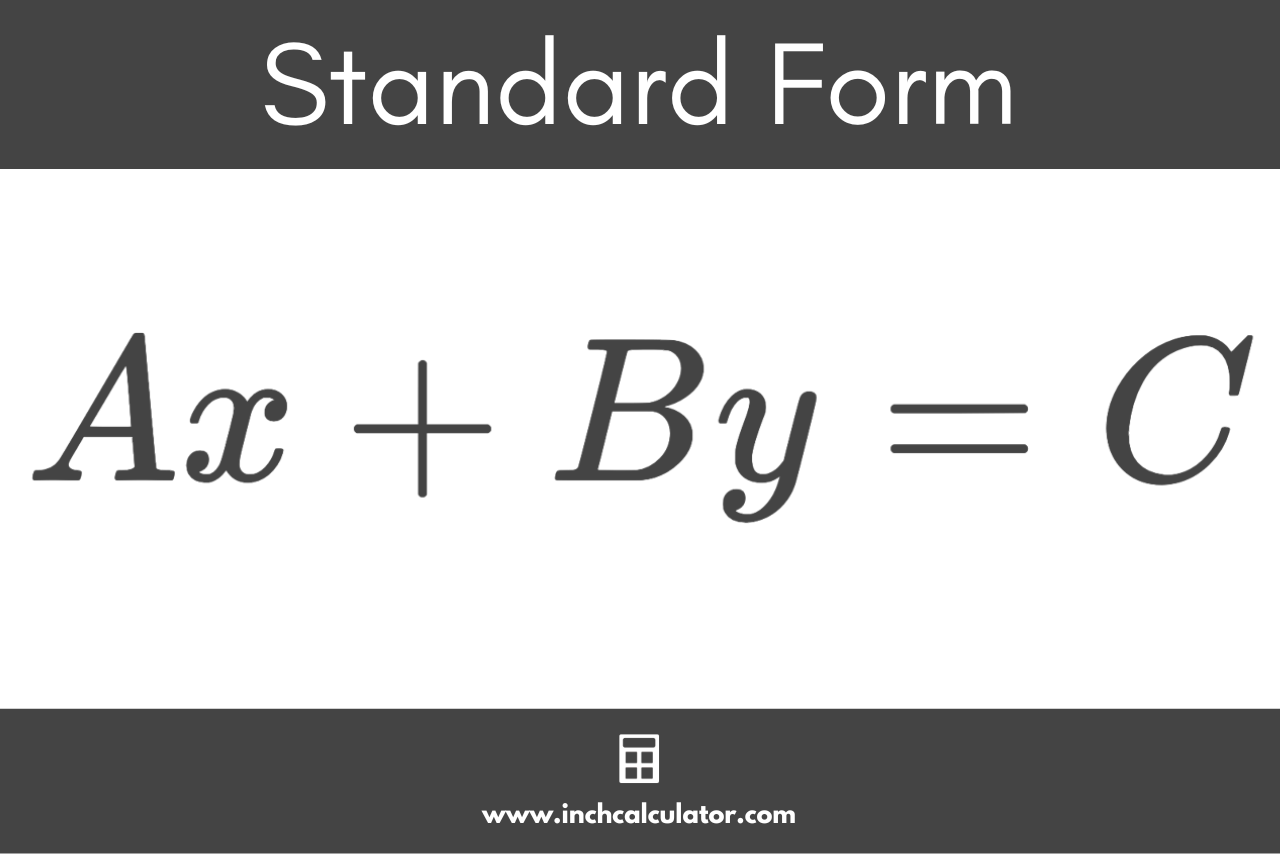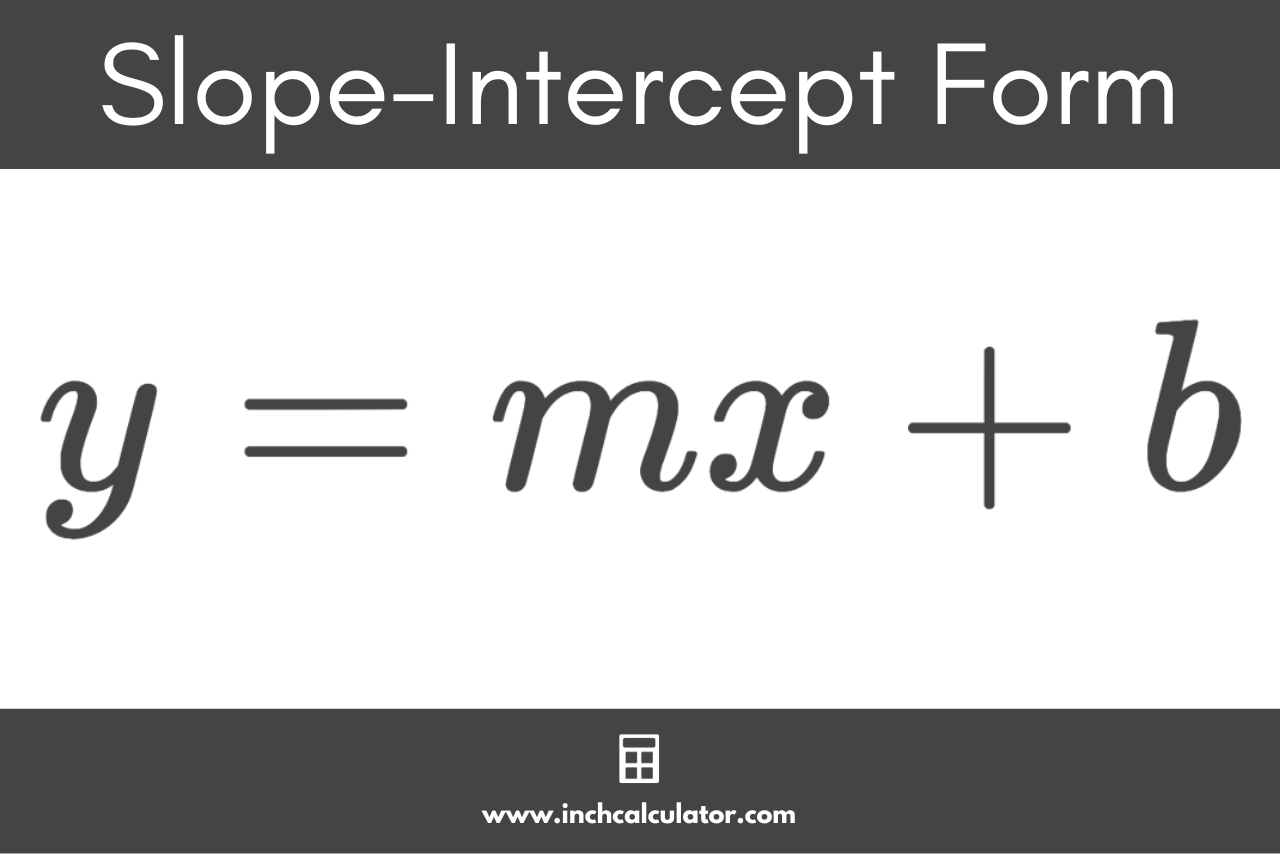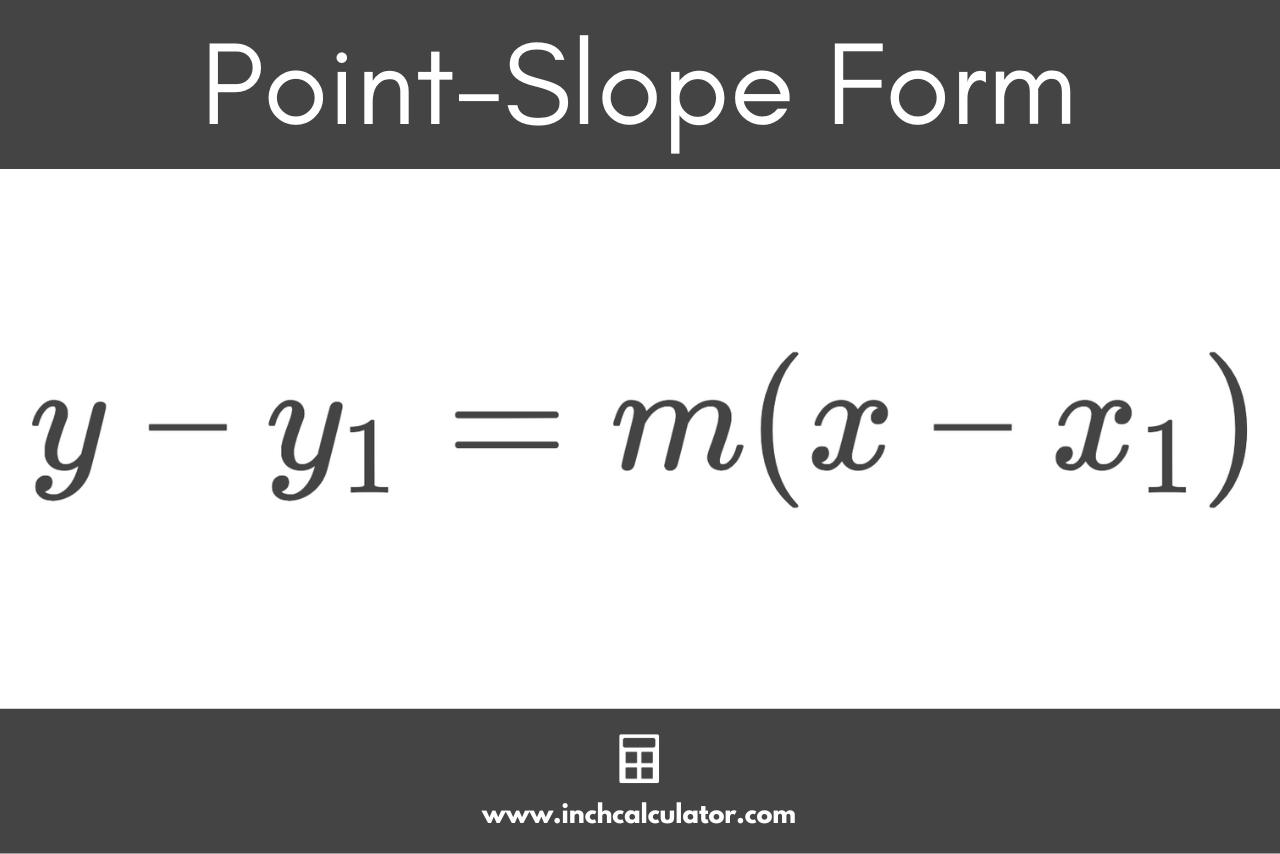# Equation of a Line Calculator

Express the equation of a line in standard form, slope-intercept form, and point-slope form using the calculator below. You can use two known points, the slope and one known point on the line, or the slope and y-intercept.

Point #1 Coordinates:
Point #2 Coordinates:
Point Coordinates:

## Line Equations:

1x - 4y = -13

### Slope-Intercept Form:

y = 1 / 4x + 3.25

### Point-Slope Form:

y − 4 = 1 / 4(x - 3)

### Line Graph:

Learn how we calculated this below

## How to Find the Equation of a Line

When working with lines, it’s possible to express the line using an equation or function or using a graph. When expressing a line using an equation, the equation allows you to find the Cartesian coordinates for any point on the line and plot it on a graph.

Equations can be used to express linear lines and also curves. However, the calculator above allows you to find the equations for linear lines.

For linear lines, there are three commonly used forms for the equation that can all be used to describe a line using its slope.

### What are the Three Line Equations?

There are three different forms of a linear equation often used to express a line; they are standard form, slope-intercept form, and point-slope form. These are different ways to describe the same line using different formats for the equation using a combination of coordinates for a point and the slope of the line.

Keep reading, and we’ll cover each of these in more detail.

#### Standard Form Equation

Standard form is the standard format for linear line equations. Standard form is a formula to express the equation of a line in a slightly different way than slope-intercept form and point-slope form, which are both covered below.

To use standard form, the values for each coefficient must be a whole number since decimals are not allowed. The coefficient for A must also be positive.

In addition to the rules above, the coefficients A, B, and C must not have any common factors other than 1.

The standard form line equation is:

Ax + By = C

The standard form equation for a linear line states that A times the x-coordinate is equal to B times the y-coordinate equals the y-intercept C.

A and B are the coefficients for the x and y coordinates of a point, and C is the y-intercept where the line crosses the y-axis.If there are any fractions in the equation, then multiply both sides by the denominator of the fractions to remove them.

This is the standard form for linear equations, but numbers can also be expressed in standard form.

#### Slope-Intercept Form Equation

Slope-intercept form is one of the most frequently used equations to represent linear lines. To use slope-intercept form, you need the slope and the y-intercept of the line.

The slope-intercept form equation is:

y = mx + b

The slope-intercept form equation for a linear line states that the y-coordinate of a point on the line is equal to the x-coordinate of the point times the slope m, plus the y-intercept b.#### Find Point-Slope Form Equation

Point-slope form is another form of equation to express a linear line. To use point-slope form, you need the slope and one point on the line.

The point-slope form equation is:

y – y1 = m(x – x1)

The point-slope form equation states that y minus y1 is equal to the slope of the line m times x minus x1.## How to Find a Line Equation Using One Point

You can write the equation of a straight line in a few steps.

### Step One: Find the Slope of the Line

The first step is to find the line’s slope, which is its steepness or gradient. If only one point is known, then you’ll need the slope, or you can use a slope calculator.

If you know the slope already, then you can skip this step.

### Step Two: Find the Y-Intercept of the Line

If you input the slope and x & y coordinates for the known point for the m, x, and y variables in the slope-intercept form equation, you can solve for the y-intercept b.

y = mx + b
y = (m × x) + b
y – b = (m × x) + b – b
y – b – y = (m × x) – y
-b = (m × x) – y
b = [(m × x) – y] × -1

You can also use a y-intercept calculator to find this.

### Step Three: Express the Line as an Equation

Finally, use the slope and y-intercept for the m and b variables in the slope-intercept form equation.

y = mx + b

The resulting equation is the slope-intercept form equation for the line.

## How to Find a Line Equation Using Two Points

If you know two points on the line, then you can also express the line as an equation.

The first step to do this is to find the slope using the slope formula:

m = (y2 – y1) / (x2 – x1)

The slope formula states that m is equal to y2 minus y1 divided by x2 minus x1. In other words, the slope is equal to the vertical change of the line divided by the horizontal change.

Input the coordinates for the two points in the slope formula, then solve for m.

After you have solved the slope, you can use it along with one of the points to find the y-intercept and express the equation of the line in slope-intercept form by following steps two and three above.

## Line Equation of a Horizontal Line

When the line is a horizontal line that is parallel to the x-axis (0° angle), it has a slope equal to 0.

When you substitute 0 for m in the slope-intercept form equation, the result looks like this:

y = 0x + b

This can be simplified to:

y = b

So, the equation of a horizontal line states that the y-coordinate is always equal to the y-intercept b, or the y-coordinate of any other point on the line.

## Line Equation of a Vertical Line

When the line is a vertical line that is perpendicular to the x-axis (90° angle), the slope is undefined. When the slope is undefined, slope-intercept form and point-slope form cannot be used to express the line.

The standard form equation for a vertical line is:

1x + 0y = C

This can be simplified to:

x = c

So, in the case of a vertical line, the equation is x is equal to c, where c is equal to the x-coordinate for any point on the line.

## References

1. IXL, Standard form of linear equations, https://www.ixl.com/math/lessons/standard-form-of-linear-equations
2. CK-12 Foundation, Linear Equations in Slope-Intercept Form, https://www.ck12.org/book/ck-12-algebra-basic/section/5.1/
3. Clapham, C., Nicholson, J., Oxford Concise Dictionary of Mathematics, 475. https://web.archive.org/web/20131029203826/http://web.cortland.edu/matresearch/OxfordDictionaryMathematics.pdf